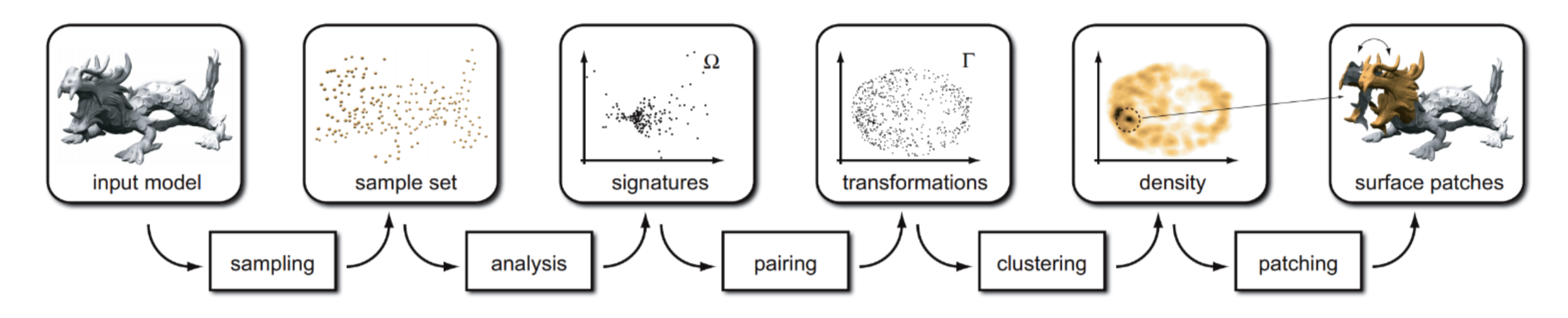# Symmetry and Orbit Detection in Point Clouds

Published:Symmetry and orbit are important global features for point clouds but the detection remains a huge challenge. In this project, I studied the paper: Symmetry and Orbit Detection via Lie-Algebra Voting. The main idea is to embedding patches into a Lie algebra $\mathfrak{sim}(d)$ with an optimal distance metric. Then RANSAC or Mean-shift is used to extract correponding structures.

In this project, I achieved the following goals:

• Adapted the algorithm to 2D point clouds and implemented it in Python, to facilitate the visualization and parameter tuning.

• Implemented the algorithm on 3d point clouds in C++, which allows a fast computation on big point cloud.

• Studied the influence of different parameters

## Requirements

### 2D Case

• >= Python 3
• Numpy, Sklearn, matplotlib

• CGAL
• Eigen
• cpp 11+